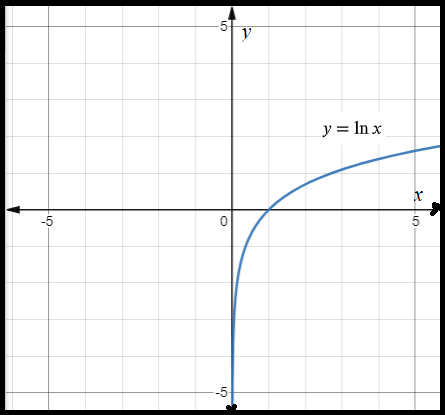1
34
views
61b
Problem

Problem 61b

Chapter 1: Functions and ModelsTextbook ExpertVerified Tutor
18 Oct 2021

Given information

The given equation is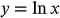.

Shift the graph of the given equation by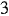units left.

Step-by-step explanation

Step 1.

Transformation rule:

The graph of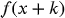is the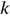units left shift of the graph of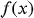Draw the graph of